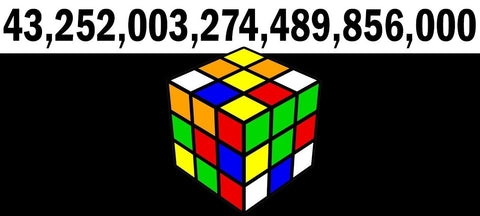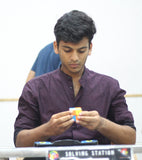## Introduction

When you ask a speedcuber how many possible combinations a speed cube has, every one of them knows this answer by heart. They all say 43 quintillions. But how many know what the exact number is? Well, that might be not relevant, yes, but how many know how or why are there 43 quintillion possibilities for a simple puzzle like a Rubik’s Cube. And in reality, how big is this number of 43 quintillions? In this article, let’s find out more about this magical number, and how extremely large this number actually is.## Why is there 43 quintillion possible combinations in a Rubik’s cube?

From the basic math we have studied in our high school, we can deduct this to be a simple case of permutations and combinations. To find the number of possible combinations, we can consider the case of assembling a cube from scratch. Let’s start with the edges. The first edge has 12 possible locations where it can be put. The 2nd edge piece can be at 11 possible locations (As 1 location is already occupied). This way we can say that the number of possible locations for the edges is 12!. Additionally, each edge can be flipped, except for the last edge (To avoid having a single flipped edge). Hence, total edge combination is 12!*211 Similarly, we can calculate the number of possible combinations for the corners, while taking care of impossible cases, which comes out to be (8!)/2 * 37 (/2 is to avoid the case of impossible 2 corner/ 2 edge flip parity). Hence, the total combination is 12! * 211 * (8!)/2 * 37, which equals 43.252 * 1018, which is 43 quintillion.

## How large is 43 quintillion as a number:

To understand how large 43 quintillion is as a number, let us consider a hypothetical case. Let us consider we have 43 quintillion A4 size papers with us, and we stack them vertically until we complete stacking up 43 quintillion papers. So we start from the ground and stack the papers on top of each other. Eventually, you find yourself to be at the height of a 3-storey building, but still, there’s more. We continue stacking and reach the height of a skyscraper. That can be approximately 150m from the ground, which is insanely tall! But we are not done yet. We keep stacking and eventually reach the height of the tallest building in the world, the size of Burj Khalifa. This is at an altitude of 830m, around six times the height of an average skyscraper. But, yes, we are not done yet! We keep going past several other peaks, like the Himalayas and the Alps and also surpassing Mt. Everest, which stands at 8.85Km. This is more than ten times the height of Burj Khalifa, and yet, we aren't even close to completion! We keep stacking, and eventually, this has to go into space! We reach a point where we get to Pluto, yet we aren't done! We keep stacking our way back to the Earth, and again, from Earth to Pluto and so on. So, to precisely complete stacking 43 quintillion A4 size papers, we would have gone from Earth to Pluto, back and forth, around 730 times! This puts the size of this number into perspective.## Conclusion:

The above hypothetical experiment puts into perspective how large the number 43 quintillion is! Seeing this small of a puzzle packing that big of many possible combinations is incredibly impressive. Essentially, all of us are trying to bring the Rubik’s cube from any of those possibilities to precisely 1 out of those 43 quintillion cases, which is the solved case. This helps us understand why solving a Rubik’s Cube is considered a big deal by a considerable part of the population and why there was a gap between the invention of the puzzle and developing a solution. So, next time if someone doesn't fully comprehend the big deal that a Rubik’s cube is, an explanation of how big 43 quintillions will suffice as a tool of humbling.Nithin Babu

Nithin Babu is the former National Record holder and National Champion in 2x2, 4x4, and 5x5 events. He started cubing at the age of 12 and has been cubing for more than a decade since 2011. His other interests include weight training and basketball, MCU, and DCEU fandom.He has attended 21 competitions and won a total of 75 podiums, including 24 gold medals. He has broken, in total, 9 National Records, and had a World Ranking of 8 in 2x2 average events by the end of 2014.